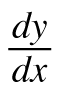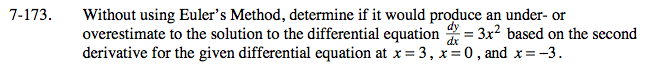### Home > CALC > Chapter 7 > Lesson 7.4.1 > Problem7-173

7-173.

Without using Euler's Method, determine if it would produce an under- or overestimate to the solution to the differential equation= 3x2 based on the second derivative for the given differential equation at x = 3, x = 0 , and x = −3. Homework Help ✎If the second derivative is positive, then tangent lines are under the curve.
If the second derivative is negative, then tangent lines are over the curve.
What does this have to do with Euler's Method?